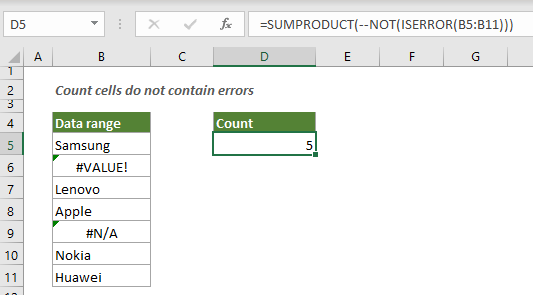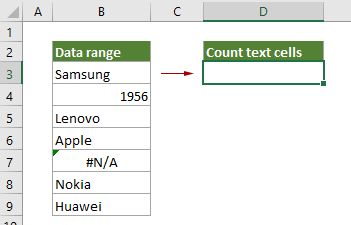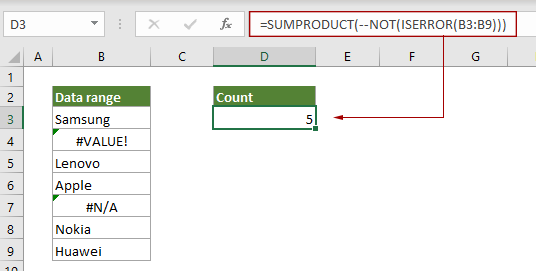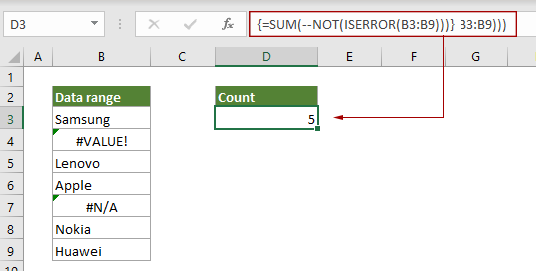## Count cells that do not contain errors in a specified range

The NOT function together with the ISERROR function can be wrapped inside the SUMPRODUCT or SUM function to count the number of cells that do not contain errors in a specified range in Excel. This tutorial provides detailed steps to help you easily get it done.Count cells that do not contain errors with SUMPODUCT function
Count cells that do not contain errors with SUM function

#### Count cells contain errors with SUMPRODUCT function

As the below screenshot shown, to count how many cells do not contain errors in B3:B9, you can do as follows.Generic Formula

=SUMPRODUCT(--NOT(ISERROR(range)))

Arguments

Range (required): The range from which you want to count cells that do not contain errors.

How to use this formula?

1. Select a blank cell such as D3 in this case.

2. Enter the below formula into it and press the Enter key to get the result.

=SUMPRODUCT(--NOT(ISERROR(B3:B9)))How these formulas work?

=SUMPRODUCT(--NOT(ISERROR(B3:B9)))

• 1) ISERROR(B3:B9): The ISERROR function returns TRUE when a cell in range B3:B9 contains an error, and returns FALSE if not. It finally returns an array {FALSE;TURE;FALSE;FALSE;TURE;FALSE;FALSE} which means that only the second and the fifth cell contain error values in the range.
• 2) NOT{FALSE;TURE;FALSE;FALSE;TURE;FALSE;FALSE}: The NOT function reverses the TURE and FALSE values in the array and returns a new array as {TURE;FALSE;TURE;TURE;FALSE;TURE;TURE}. On the contrary, each TURE value in this new array indicates a cell that does not contain an error value.
• 3) --{TURE;FALSE;TURE;TURE;FALSE;TURE;TURE}: These two minus signs convert “TRUE” into 1 and “FALSE” into 0. Here you will get a new array as {1;0;1;1;0;1;1}
• 4) SUMPRODUCT{1;0;1;1;0;1;1}: The SUMPRODUCT function sums all numbers in the array and returns the final result as 5.

#### Count cells contain errors with SUM function

The NOT function together with the ISERROR function can be wrapped inside the SUM function to count the number of cells that do not contain errors in a specified range in Excel.Generic Formula

{=SUM(--NOT(ISERROR(range))}

Arguments

Range (required): The range from which you want to count cells that do not contain errors.

Note: This formula must be entered as an array formula. After applying the formula, if there are curly brackets wrapped around the formula, an array formula is successfully created.

How to use this formula?

1. Select a blank cell to output the result.

2. Enter the below formula into it, and then press the Ctrl + Shift + Enter keys simultaneously to get the result.

=SUM(--NOT(ISERROR(B3:B9)))How these formulas work?

=SUM(--NOT(ISERROR(B3:B9)))

• 1) ISERROR(B3:B9): The ISERROR function returns TRUE when a cell in range B3:B9 contains an error, and returns FALSE if not. It finally returns an array {FALSE;TURE;FALSE;FALSE;TURE;FALSE;FALSE} which means that only the second and the fifth cell contain error values in the range.
• 2) NOT{FALSE;TURE;FALSE;FALSE;TURE;FALSE;FALSE}: The NOT function reverses the TURE and FALSE values in the array and returns a new array as {TURE;FALSE;TURE;TURE;FALSE;TURE;TURE}. On the contrary, each TURE value in this new array indicates a cell that does not contain an error value.
• 3) --{TURE;FALSE;TURE;TURE;FALSE;TURE;TURE}: These two minus signs convert “TRUE” into 1 and “FALSE” into 0. Here you will get a new array as {1;0;1;1;0;1;1}
• 4) SUM{1;0;1;1;0;1;1}: The SUM function sums all numbers in the array and returns the final result as 5.

Note: Both the ISERROR function and the ISERR function can be used to check for error values in a range of cells. So, you can replace the ISERROR with the ISERR function in above formulas to count non error cells in a specified range as well. However, it should be noted that the ISERR function ignores the N/A error and regards it as a normal value in the result.

#### Related functions

Excel ISERROR function
The ISERROR function checks whether a value is an error and returns TRUE or FALSE.

Excel SUMPRODUCT function
In Excel, the SUMPRODUCT function can be used to multiply two or more columns or arrays together, and then get the sum of products.

Excel SUM unction

#### Related formulas

Count The Number Of Text Cells
To count the number of cells that contain text in a specified range, the COUNTIF function can help to easily get it done. Here this article will introduce the formula in details to help you solve the problem.

Count number of cells that do not contain many values
This post will step through how to count number of cells that do not contain many values in a specified range in Excel.

Count number of date cells in a range by day of week
Excel provides the combination of SUMPRODUCT and WEEKDAY functions to help us easily count the number of specified weekdays within a range. This tutorial provides a step by step guide to help you get through it.

Count cells that do not contain specific text
This tutorial provides detailed steps to help counting cells that do not contain specific text in Excel.

### The Best Office Productivity Tools

#### Kutools for Excel - Helps You To Stand Out From Crowd

Would you like to complete your daily work quickly and perfectly? Kutools for Excel brings 300 powerful advanced features (Combine workbooks, sum by color, split cell contents, convert date, and so on...) and save 80% time for you.

• Designed for 1500 work scenarios, helps you solve 80% Excel problems.
• Reduce thousands of keyboard and mouse clicks every day, relieve your tired eyes and hands.
• Become an Excel expert in 3 minutes. No longer need to remember any painful formulas and VBA codes.
• 30-day unlimited free trial. 60-day money back guarantee. Free upgrade and support for 2 years.#### Office Tab - Enable Tabbed Reading and Editing in Microsoft Office (include Excel)

• One second to switch between dozens of open documents!
• Reduce hundreds of mouse clicks for you every day, say goodbye to mouse hand.
• Increases your productivity by 50% when viewing and editing multiple documents.
• Brings Efficient Tabs to Office (include Excel), Just Like Chrome, Firefox, And New Internet Explorer.